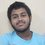# Proving a floor sum.

Given that $$a,b\in\Bbb{Z}^+$$ and $$\gcd(a,b)=1$$.

Prove the following:

$\left\lfloor\frac{a}{b}\right\rfloor+\left\lfloor2\cdot\frac{a}{b}\right\rfloor+\left\lfloor3\cdot\frac{a}{b}\right\rfloor+\ldots+\left\lfloor(b-1)\cdot\frac{a}{b}\right\rfloor=\frac{(a-1)(b-1)}{2}$

Clarifications:

• $\lfloor\cdot\rfloor$ denotes the floor function (greatest integer function).
• $\gcd(x,y)$ denotes the greatest common divisor of $x$ and $y$.

Hint:

It'd be helpful to prove this first before attempting to prove the main problem.

$\forall~a,b\in\Bbb{Z}^+\mid\gcd(a,b)=1\implies n\frac{a}{b}\not\in\Bbb{Z}~~\forall~~0\lt n\lt b~\land~n\in\Bbb{Z}$Note by Prasun Biswas
6 years, 2 months ago

This discussion board is a place to discuss our Daily Challenges and the math and science related to those challenges. Explanations are more than just a solution — they should explain the steps and thinking strategies that you used to obtain the solution. Comments should further the discussion of math and science.

When posting on Brilliant:

• Use the emojis to react to an explanation, whether you're congratulating a job well done , or just really confused .
• Ask specific questions about the challenge or the steps in somebody's explanation. Well-posed questions can add a lot to the discussion, but posting "I don't understand!" doesn't help anyone.
• Try to contribute something new to the discussion, whether it is an extension, generalization or other idea related to the challenge.

MarkdownAppears as
*italics* or _italics_ italics
**bold** or __bold__ bold
- bulleted- list
• bulleted
• list
1. numbered2. list
1. numbered
2. list
Note: you must add a full line of space before and after lists for them to show up correctly
paragraph 1paragraph 2

paragraph 1

paragraph 2

[example link](https://brilliant.org)example link
> This is a quote
This is a quote
    # I indented these lines
# 4 spaces, and now they show
# up as a code block.

print "hello world"
# I indented these lines
# 4 spaces, and now they show
# up as a code block.

print "hello world"
MathAppears as
Remember to wrap math in $$ ... $$ or $ ... $ to ensure proper formatting.
2 \times 3 $2 \times 3$
2^{34} $2^{34}$
a_{i-1} $a_{i-1}$
\frac{2}{3} $\frac{2}{3}$
\sqrt{2} $\sqrt{2}$
\sum_{i=1}^3 $\sum_{i=1}^3$
\sin \theta $\sin \theta$
\boxed{123} $\boxed{123}$

Sort by:

Use the fact that $\lfloor x\rfloor=x-\{x\}$ to rewrite as

$\sum_{k=1}^{b-1}\left\lfloor\dfrac{ka}{b}\right\rfloor=\dfrac a b\sum_{j=1}^{b-1} j-\sum_{k=1}^{b-1}\left\{\dfrac{ka}{b}\right\}=\dfrac{a(b-1)}{2}-\sum_{k=1}^{b-1}\left\{\dfrac{ka}{b}\right\}.$

Now

$\sum_{k=1}^{b-1}\left\{\dfrac{ka}{b}\right\}=\sum_{k=1}^{(b-1)/2}\left(\left\{\dfrac{ka}{b}\right\}+\left\{\dfrac{(b-k)a}{b}\right\}\right)=\sum_{k=1}^{(b-1)/2}\left(\left\{\dfrac{ka}{b}\right\}+\left\{a-\dfrac{ka}{b}\right\}\right).$

Here no term can be zero since $na/b$ is not integer for $1\le n provided that $(a,b)=1$. So $\forall k\in[1, (b-1)/2]$

$\left\{\dfrac{ka}{b}\right\}+\left\{a-\dfrac{ka}{b}\right\}=1\implies \sum_{k=1}^{(b-1)/2}\left(\left\{\dfrac{ka}{b}\right\}+\left\{a-\dfrac{ka}{b}\right\}\right)=\sum_{k=1}^{(b-1)/2}1=\dfrac{b-1}{2}$

and we're, um, done.

- 6 years, 2 months ago

Very well done....

- 6 years, 2 months ago

Consider $b=8$ and $a=9$. We have $\gcd(a,b)=1$ but $\frac{(b-1)}{2}$ is not a positive integer $\geq 1$. Then, what does the sum $\displaystyle\sum_{k=1}^{(b-1)/2}(1)$ mean?

- 6 years, 2 months ago

The sum is to $b-1$, as in $\lfloor (b-1) \frac{ a}{b} \rfloor$, and not to $\frac{ b-1}{2}$ as you had it.

Staff - 6 years, 2 months ago

Warning: there is a huge hint below.

$na (\mod b)$ is pairwise distinct for $n=1, 2, ..., b$. Proof: The opposite is false by Pigeonhole Principle. Hence if we limit $n$ to $1, 2, 3, ..., b-1$ the possible remainders are $1, 2, ..., b-1$, once each.

From here we can find the sum of the $b-1$ fractional parts of $n•\frac {a}{b}, n=1, 2, ..., b-1$. We know their sum, so we know the sum of their floors.

- 6 years, 2 months ago

There's an easier approach (though essentially based on the same idea).